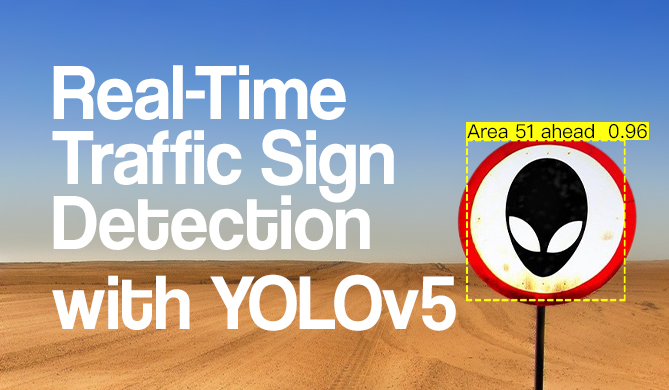Comparing Datetime with Delorean in Python - With and Without Timezones

# Comparing Datetime with Delorean in Python - With and Without Timezones### Introduction

Working with datetime can be a challenging, and handling datetime in Python is no exception. Python's built-in datetime module introduced us to several classes - date, datetime, time, timezone and timedelta, and several external libraries have spawned to address the issues present in the official module, such as Arrow.

In this guide, we'll take a look at how to compare datetime objects in Delorean - a library built upon pytz which is the standard library for solving problems relating to timezones, and dateutil which is used to calculate deltas between any 2 given datetime objects.

### Installing Delorean Setting Up a Virtual Environment

Delorean is available for installation via pip. It is generally advisable to work in a virtual environment because it enables you to organize dependencies required by different projects isolated.

On Linux,or MacOS to create a virtual environment, we'd run:

$python3 -m venv env$ source env/bin/activate
$python3 -m pip install delorean  Alternatively, on Windows we can run: $ virtualenv env
$.\env\Scripts\activate$ python3 -m pip install delorean


### Comparing Datetime without Timezone (Naive Datetime) Using Delorean

Let's start out by comparing timezone-naive dates - dates that don't carry timezone information, but represent a given point in time. That being said - you can set the timezone of a Delorean object but, when printed back, it'll be in UTC:

from delorean import Delorean
import datetime

naive_d1 = Delorean(datetime=datetime.datetime(2021, 5, 15), timezone='UTC').naive
naive_d2 = Delorean(datetime=datetime.datetime(2021, 5, 15, 5), timezone='UTC').naive
naive_d3 = Delorean(datetime=datetime.datetime(2021, 5, 15, 5), timezone='US/Central').naive

print("naive_d1 == naive_d2 is", naive_d1 == naive_d2)
print("naive_d2 > naive_d1 is", naive_d2 > naive_d1)
print("naive_d3 == naive_d2 + 5h is", naive_d3 == naive_d2 + datetime.timedelta(hours=5))


This results in:

naive_d1 == naive_d2 is False
naive_d2 > naive_d1 is True
naive_d3 == naive_d2 + 5h is True


naive_d1 is not equal to naive_d2 because it has more information - the hours are defined, and thus aren't default (noon).

This makes naive_d2 "greater" than naive_d1, being at a later point in time.

naive_d3 is in a different timezone than naive_d2, but this is easily rectified by simply adding a timedelta of 5 hours to naive_d2 to match them, and True is returned for their equality.

If you were to print out naive_d2 and naive_d3, even though they were both set to 5AM:

print(naive_d2)
print(naive_d3)


You'd get a shifted result, since they're both matched to UTC:

2021-05-15 05:00:00
2021-05-15 10:00:00


### Comparing Datetime with Timezone (Aware Datetime) Using Delorean

Timezone aware dates aren't matched onto a single timezone. If you were to make two dates, denoting the same clock time in two different timezones:

d1 = Delorean(datetime=datetime.datetime(2021, 5, 15, 5), timezone='UTC')
d2 = Delorean(datetime=datetime.datetime(2021, 5, 15, 5), timezone='Africa/Lagos')


If you were to print them out - they'd both be denoting 5AM, but in different timezones:

print(d2)
print(d3)


This results in:

Delorean(datetime=datetime.datetime(2021, 5, 15, 5, 0), timezone='UTC')
Delorean(datetime=datetime.datetime(2021, 5, 15, 5, 0), timezone='Africa/Lagos')


## Free eBook: Git Essentials

Check out our hands-on, practical guide to learning Git, with best-practices, industry-accepted standards, and included cheat sheet. Stop Googling Git commands and actually learn it!

This makes the comparison a bit different than naive dates - is d2 equal to d3 here? They're both denoting the same clock time, but not the same point in time. Delorean will compare whether two Delorean objects denote the same point in time, so they're not the same in the library's eyes.

You can add time to timezoned dates to match them, though. The Africa/Lagos timezone is just an hour behind of UTC so if we add an hour to it, they'll denote the same point in time:

from delorean import Delorean
import datetime

d1 = Delorean(datetime=datetime.datetime(2021, 5, 15), timezone='UTC')
d2 = Delorean(datetime=datetime.datetime(2021, 5, 15, 5), timezone='UTC')
d3 = Delorean(datetime=datetime.datetime(2021, 5, 15, 5), timezone='Africa/Lagos')

print("d2 == d3 +1h is", d2 == d3 + datetime.timedelta(hours=1))
print("d1 > d3 -", d1 > d3)


Additionally, since d1 doesn't have data on hours - it's set to noon. Even though d1 belongs to UTC, it's still 4 hours before d3:

d2 == d3 +1h is True
d1 > d3 - False


### Conclusion

In this guide, we've taken a look at how to compare timezone-naive and timezone-aware dates in Delorean.

Last Updated: August 23rd, 2021

Get tutorials, guides, and dev jobs in your inbox.

Geoffery, JosephAuthor

I am a software developer with interests in open source, android development (with kotlin), backend web development (with python), and data science (with python as well).Project

### Real-Time Road Sign Detection with YOLOv5

# python# machine learning# computer vision# pytorch

If you drive - there's a chance you enjoy cruising down the road. A responsible driver pays attention to the road signs, and adjusts their...David Landup
DetailsProject

### Data Visualization in Python: The Collatz Conjecture

# python# matplotlib# data visualization

The Collatz Conjecture is a notorious conjecture in mathematics. A conjecture is a conclusion based on existing evidence - however, a conjecture cannot be proven....Details